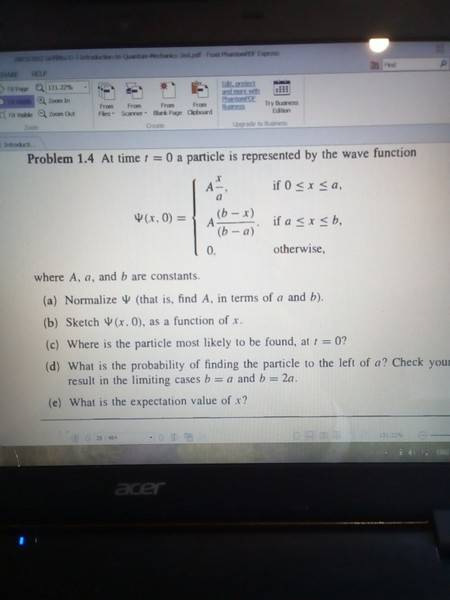# Most likely location of a particle versus expected value of

Hello. I'm using Griffiths' introductory textbook on quantum mechanics, which I have just begun, and have arrived at a question on a simple wave function:The answer to the first question I know to be √(3/b).

The answer to part e is (2a + b) / 4.

My problem is that I don't understand how there is any difference between the most likely position of the particle on the X axis and its expected value, given above for part e.

I have checked the solution manual and its its answer to part c was just 'a', which was my initial instinct, because the graph reaches its maximum at a. But this seems to me to be contrary to the notion of an expected value which, among other things, in a probability context should be the most likely value.

Has the solutions manual got it wrong, or have I?

#### Attachments

DrClaude
Mentor
In the case of a normal distribution, the most probable value is the same as the expectation value, but this is not the case for most distributions.

If you look at the Wikipedia page on multimodal distribution, you will see figures where this is obvious: when you have two humps more or less symmetrical, the most probable value will be at the top of one of the bumps but the expectation value near the middle of the two bumps.

(Note: by definition, the most probable value is the value where the probability distribution is the highest.)

•Prometheus18
PeroK
Homework Helper
Gold Member
2021 Award
Hello. I'm using Griffiths' introductory textbook on quantum mechanics, which I have just begun, and have arrived at a question on a simple wave function:

View attachment 231470

The answer to the first question I know to be √(3/b).

The answer to part e is (2a + b) / 4.

My problem is that I don't understand how there is any difference between the most likely position of the particle on the X axis and its expected value, given above for part e.

I have checked the solution manual and its its answer to part c was just 'a', which was my initial instinct, because the graph reaches its maximum at a. But this seems to me to be contrary to the notion of an expected value which, among other things, in a probability context should be the most likely value.

Has the solutions manual got it wrong, or have I?

In general, the expected value is the mean (or average) and the most likely is called the mode. As a simple example, the expected value of the roll of a die is 3.5, although all values from 1-6 are equally likely. And, of course, you can't actually ever throw a 3.5. Sometimes, therefore, the expected value is not a possible or valid outcome.

•Prometheus18 and DrClaude
DrClaude
Mentor
Sometimes, therefore, the expected value is not a possible or valid outcome.
Good point. This can be the case in quantum mechanics, where you for instance have spin-1/2 in states such that ##\langle S_z \rangle = 0## or ##\langle S_z \rangle = -\hbar/4##, while you can only ever measure ##\hat{S}_z## as ##\hbar/2## or ##-\hbar/2##.

•PeroK
Yes, thanks both of you. I've just been having a slightly dull morning; I should know the difference between a mode and mean by now!

•PeroK